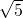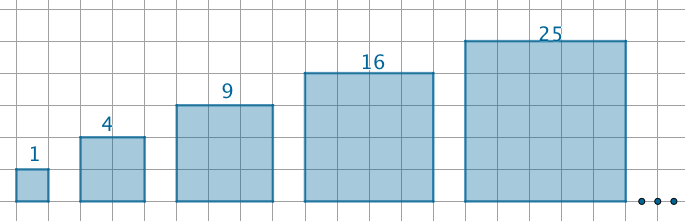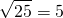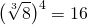The idea of powers and roots has been around for a very long time, but the way we write them down and communicate them has changed over the years. You can see from this table, called ‘the origins of some mathematical symbols‘ that the square root symbol,has been around since 1524; and exponents as we now use them showed up 100 years later, they have been around since 1637.

We now use both notations interchangeably, but one can note a preference for root notation in geometry and for exponent notation in calculus.

Let’s see how they both say the same thing!

We know thatbecause.

We can visualize it by looking at a square of side length, which is why we call these numbers ‘square numbers’:Another way to state this relationship betweenandis to sayWhat we mean byis the number, when multiplied by itself, gives us.This relationship is relatively easy to think about because the missing number is an integer.That number turns out to beThe decimals go on and on. It is an irrational number, and slighty more difficult to think about. To make it easier to write down, we generally leave it as a symbol:# Exponents

Now, returning to exponents, we know the law that says.

We also know thatSo, we can write that:.

Note that:andIn other words,andare the same number. You can type them both on your calculator and get the same answer.

Extending the idea, we haveIn other words,And in general:# What about a number that has a power and a root?

Finally, let’s think about a number such asorWhich means

‘take the number 8, raise it to the power of 4 then take the cube root’ or…

‘take the number 8, take the cube root, then raise to the power of 4’.

It’s easier to do the second calculation – but you can check on a calculator that they reach the same conclusion.Therefore,Now, as an exponent, the cube root is the same as the exponent, the first part reads:The second part is, ‘raise to the power 4’:Now, their is an exponent law that reminds us what do to here:. So we can write:When it comes to converting from root notation to exponent notation or vice versa:In general:# 2 to the power of 3.5

What is the meaning of? We can see the value is approximately 11, and it lies evenly on the graph of, between the pointsand.

Let’s convert the decimal to a fraction:Therefore,As every decimal that either terminates or repeats can be written as a fraction, we can evaluate or give meaning to every rational (fractional) exponent in a similar way.

Top